##### Algebra I Workbook For Dummies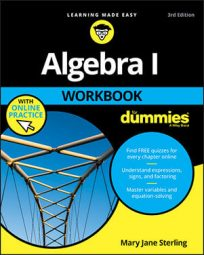Formulas, patterns, and procedures used for simplifying expressions and solving equations are basic to algebra. Use the equations, shortcuts, and formulas you find for quick reference. This Cheat Sheet offers basic information and short explanations (and some words of advice on traps to avoid).

## Order of operations in algebra

When creating simpler and more useful expressions, you want to be careful not to change the original value. By applying the order of operations, you maintain that value.

Apply the order of operations when no grouping symbols, such as parentheses, interrupt. When more of one level occurs in a problem, do them in order from left to right. When you perform operations on algebraic expressions and you have a choice between one or more operations to perform, use the following order:

1. Powers and roots

2. Multiplication and division

These rules are interrupted if the problem has grouping symbols. You first need to perform operations in grouping symbols, such as ( ), { }, [ ] , above and below fraction lines, and inside radicals.

## Rules of exponents

Exponents are shorthand for repeated multiplication. The rules for performing operations involving exponents allow you to change multiplication and division expressions with the same base to something simpler. Remember that in xa the x is the base and the “a�? is the exponent.

Assume that neither x nor y are equal to zero: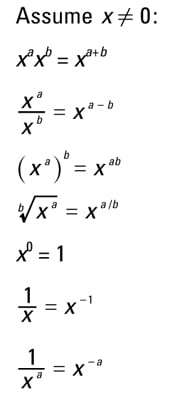## Selected math formulas step by step

Algebraic formulas make life (and algebra) simpler. You save time by not having to perform more complicated tasks. When using the formulas, use the appropriate rules for simplifying algebraic expressions. Also watch out for pitfalls; to help you, an asterisk (*) appears beside steps where errors are easy to make.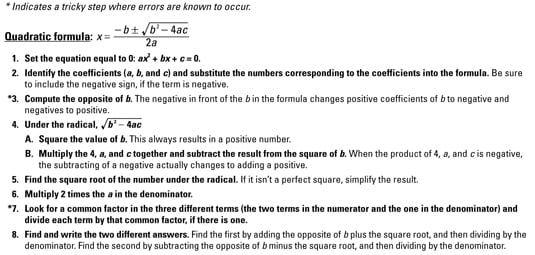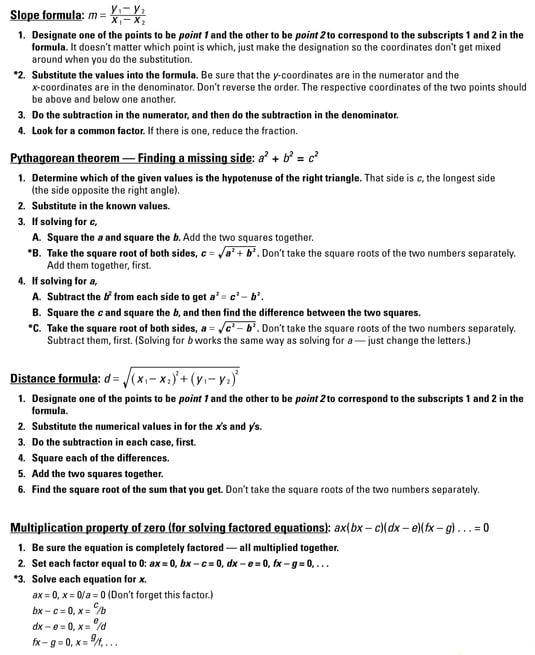## Factoring special problems

Binomials, their powers, and their products with selected trinomials occur frequently in algebraic processes. By using the patterns shown here, you save time and reduce the opportunity for errors.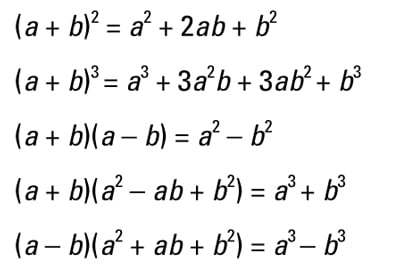## Formulas for common geometric shapes

Depending on the algebra problem, you’ll need to know some geometry. The following represents some of the most common shapes in geometry and their formulas for perimeter, area, volume, surface areas, and circumference:

Two-Dimensional Shapes
Shape Perimeter/Circumference Area
Rectangle P = 2(l + w) A = lw
Square P = 4s A = s2
Triangle P = a + b + c A = 1/2bh
Trapezoid P = a + b1 + c + b2 A = 1/2h(b1 + b2)
Isosceles Trapezoid P = 2w + b1 + b2 A = 1/2h(b1 + b2)
Circle C = πd = 2 π r A = π r2
Three-Dimensional Shapes
Shape Surface Area Volume
Box SA = 2lw + 2lh + 2wh V = lwh
Sphere SA = 4 π r2 V = 4/3 π r3
Cylinder SA = 2 π r(r + h) V = π r2h